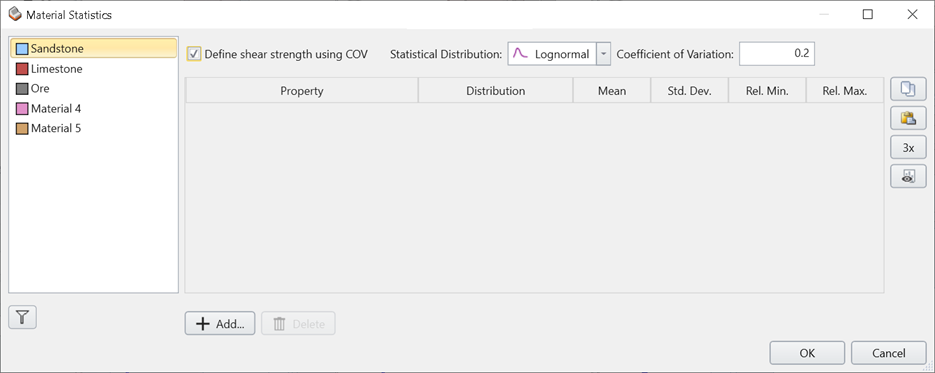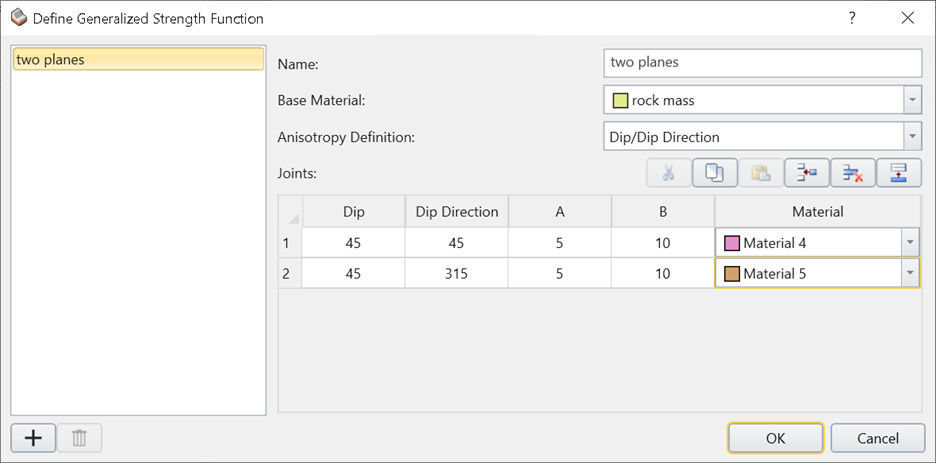The Rocscience International Conference 2021 Proceedings are now available. Read Now

# Probabilistic Shear Strength

In Slide3 the user has the option to consider material variability either by adding random variables for individual material properties (e.g. cohesion, friction angle, etc.), or by adding a random variable to consider the shear strength of the material.

The advantage of considering the shear strength as a random variable is that you only have to define it once, instead of several times for the different material properties. Additionally, the probabilistic shear strength option can be used on all material strength types, including Shear/Normal and Discrete Strength.

## Enabling Probabilistic Shear Strength

To use the option, the user must first turn on Probabilistic Analysis from Analysis > Project Settings > Statistics as usual.

Then in the enabled Statistics menu, select: Statistics > Material Statistics. The user must then check the “Define shear strength using COV” box as shown:This will enable the Statistical Distribution and Coefficient of Variation inputs. The distribution options are the same as the ones found throughout the program. The coefficient of variation is defined as (standard deviation/mean). Since the shear strength value is different at different locations in the slope, this allows the user to define the distribution without needing to know the mean value, but only as a ratio of the mean. The COV input is enabled for Lognormal, Normal, and Beta distributions.

The Exponential, Uniform, and Triangular distributions have a range input instead. To use the range input, keep in mind that the default value is 1.0, meaning that the shear strength in the slope is multiplied by 1.0 in the deterministic case. You can change this multiplier to be uniform and ranging from 0.7 and 1.3 for example. This means that you will define a uniform distribution between 0.7 and 1.3, and that the shear strength will be multiplied with values from this range in each simulation. The same idea applies to the triangular and exponential distributions.

Regardless of the distribution selected, after computing the user can plot the histogram of samples using Statistics > Histogram. Here they will be able to see a histogram of the shear strength factor applied to each sample, which will always have a mean of 1.0 (i.e. no factor is applied).

# Note on Generalized Anisotropic Strength

The probabilistic shear strength option can be used on any material strength type, including Generalized Anisotropic. However, an important note is warranted here: consider the case where the user has defined a Generalized Anisotropic material that uses the strengths of rock mass, Material 4 and Material 5 as shown:The user can then define probabilistic shear strength for the Generalized Anisotropic material, as well as for rock mass, Material 4, and 5. However, the distribution of the Generalized Anisotropic material will be independent of those of the three materials included, meaning that the distributions of rock mass, Material 4, and 5 are not derived from the Generalized Anisotropic distribution.